# NCERT Solutions for Chapter 13 Symmetry Class 6 Maths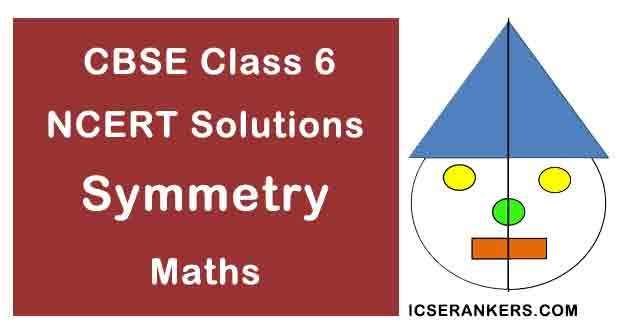Chapter Name Symmetry NCERT Solutions Class CBSE Class 6 Textbook Name Maths Related Readings NCERT Solutions for Class 6NCERT Solutions for Class 6 Maths Summary of Symmetry

Exercise 13.1

Question 1: List any four symmetrical from your home or school.

Solution

Notebook, Blackboard, Glass, Inkpot.

Question 2: For the given figure, which one is the mirror line, l1 or l2 ?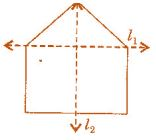Solution

l2 is the mirror line as both sides of the lines are symmetric.

Question 3: Identify the shapes given below. Check whether they are symmetric or not. Draw the line of symmetry as well.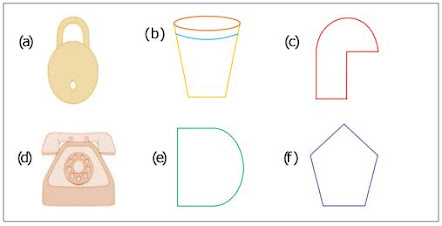Solution

(a) Symmetric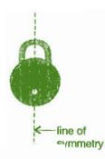(b) Symmetric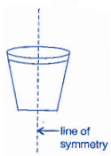(c) Not symmetric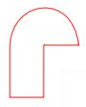(d) Symmetric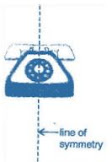(e) Symmetric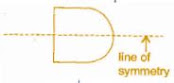(f) Symmetric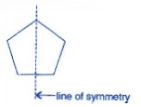Question 4: Copy the following on a square paper. A square paper is what you would have used in your arithmetic notebook in earlier classes. Then complete them such that the dotted line is the line of symmetry.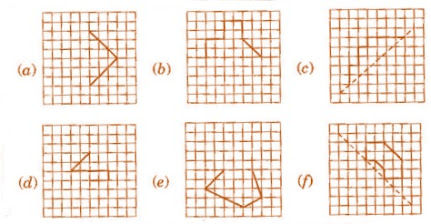Solution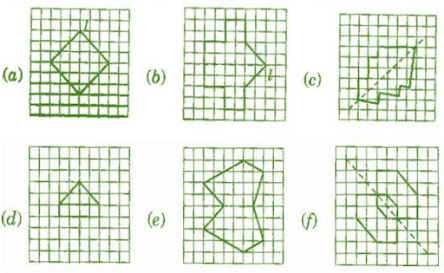Question 5: In the figure, l is the line of symmetry. Complete the diagram to make it symmetric.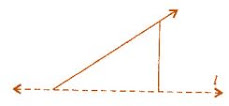Solution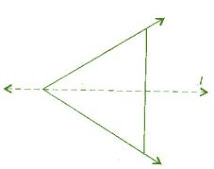Question 6: In the figure, l is the line of symmetry. Draw the image of the triangle and complete the diagram, so that it becomes symmetric.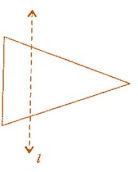Solution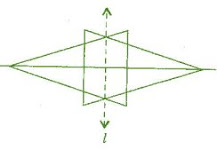Exercise 13.2

Question 1: Find the number of lines of symmetry for each of the following shapes: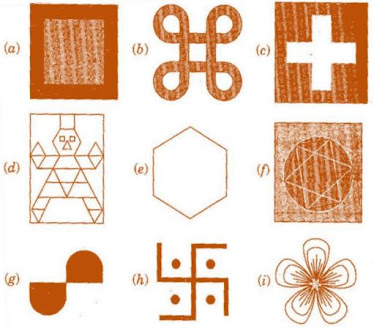Solution

(a) 4

(b) 4

(c) 4

(d) 1

(e) 6

(f) 4

(g) 0

(h) 0

(i) 3

Question 2: Copy the triangle in each of the following figures on squared paper. In each case, draw the line(s) of symmetry, if any and identify the type of triangle. (Some of you may like to trace the figures and try paper-folding first!)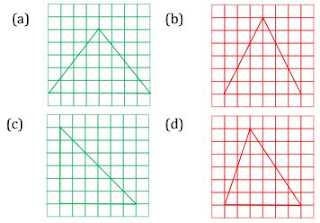Solution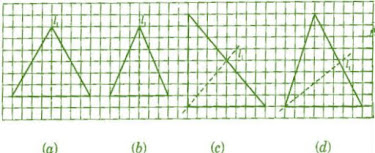(a) l1 is the line of symmetry.

(b) l1 is the line of symmetry.

(c) l1 is the line of symmetry.

(d) No line of symmetry.

Question 3: Complete the following table: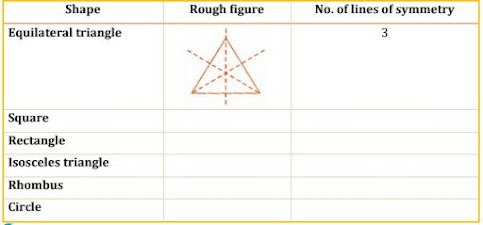SolutionQuestion 4: Can you draw a triangle which has:

(a) exactly one line of symmetry?

(b) exactly two lines of symmetry?

(c) exactly three lines of symmetry?

(d) no lines of symmetry?

Sketch a rough figure in each case.

Solution

(a) Yes, Isosceles triangle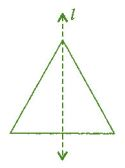(b) No such triangle cannot be formed.

(c) Yes, Equilateral triangle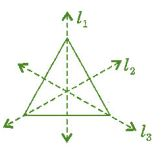(d) Yes, Scalene triangle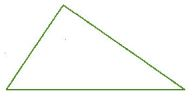Question 5: On a squared paper, sketch the following:

(a) A triangle with a horizontal line of symmetry but no vertical line of symmetry.

(b) A quadrilateral with both horizontal and vertical lines of symmetry.

(c) A quadrilateral with a horizontal line of symmetry but no vertical line of symmetry.

(d) A hexagon with exactly with two lines of symmetry.

(e) A hexagon with six lines of symmetry.

(Hint: It will be helpful if you first draw the lines of symmetry and then complete the figures)

Solution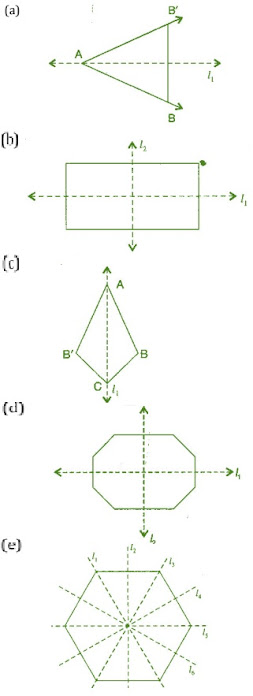Question 6: Trace each figure and draw the lines of symmetry, if any: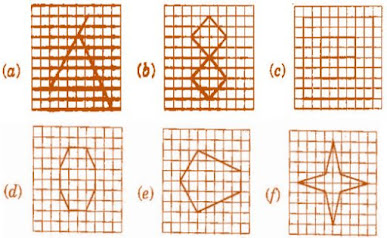Solution

(a) No line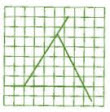(b) Two lines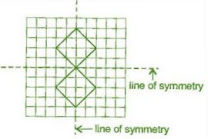(c) Four lines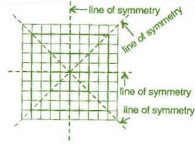(d) Two lines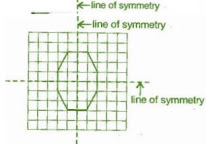(e) One line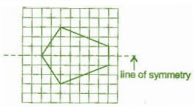(f) Four lines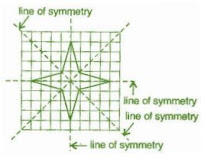Question 7: Consider the letters of English alphabets A to Z. List among them the letters which have:

(a) vertical lines of symmetry (like A)

(b) horizontal lines of symmetry (like B)

(c) no lines of symmetry (like Q)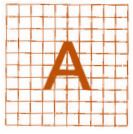Solution

Vertical lines: A, H, I, M, O, T, U, V, W, X, Y

Horizontal lines: B, C, D, E, H, I, K, O, X

No line of symmetry: F, G, J, N, P, Q, R, S, Z

Question 8: Given here are figures of a few folded sheets and designs drawn about the fold. In each case, draw a rough diagram of the complete figure that would be seen when the design is cut off.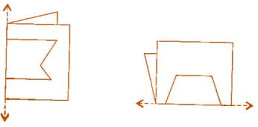Solution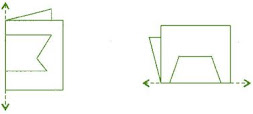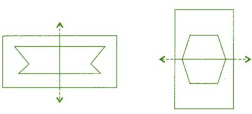Exercise 13.3

Question 1: Find the number of lines of symmetry in each of the following shapes. How will you check your answer?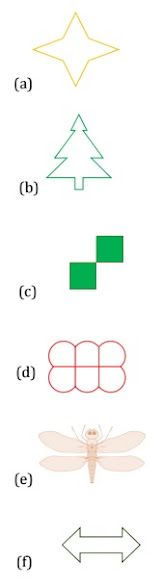Solution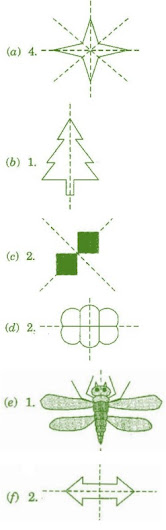Question 2: Copy the following drawing on squared paper. Complete each one of them such that the resulting figure has two dotted lines as two lines of symmetry.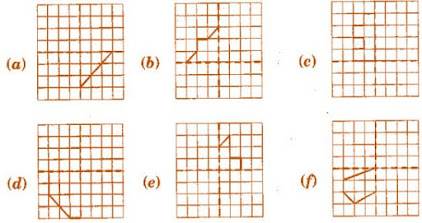How did you go about completing the picture?

Solution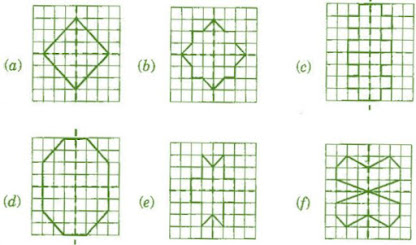Question 3: In each figure below, a letter of alphabet is shown along with a vertical line. Take the mirror image of the letter in the given line. Find which letters look the same after reflection (i.e., which letters look the same in the image) and which do not. Can you guess why?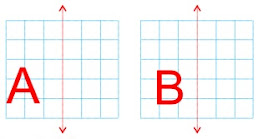try for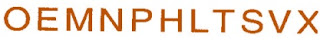Solution

Same after reflection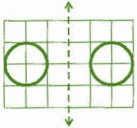Different after reflection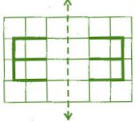Same after reflection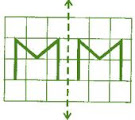Different after reflection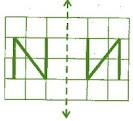Different after reflection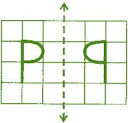Same after reflectionDifferent after reflection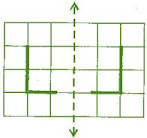Same after reflection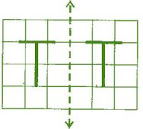Different after reflection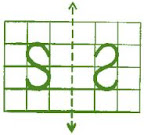Same after reflection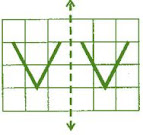Same after reflection